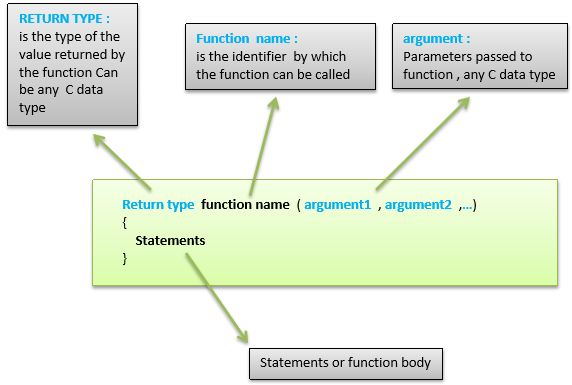# Arduino - Functions

Functions allow structuring the programs in segments of code to perform individual tasks. The typical case for creating a function is when one needs to perform the same action multiple times in a program.

Standardizing code fragments into functions has several advantages −

• Functions help the programmer stay organized. Often this helps to conceptualize the program.

• Functions codify one action in one place so that the function only has to be thought about and debugged once.

• This also reduces chances for errors in modification, if the code needs to be changed.

• Functions make the whole sketch smaller and more compact because sections of code are reused many times.

• They make it easier to reuse code in other programs by making it modular, and using functions often makes the code more readable.

There are two required functions in an Arduino sketch or a program i.e. setup () and loop(). Other functions must be created outside the brackets of these two functions.

The most common syntax to define a function is −## Function Declaration

A function is declared outside any other functions, above or below the loop function.

We can declare the function in two different ways −

The first way is just writing the part of the function called a function prototype above the loop function, which consists of −

• Function return type
• Function name
• Function argument type, no need to write the argument name

Function prototype must be followed by a semicolon ( ; ).

The following example shows the demonstration of the function declaration using the first method.

### Example

```int sum_func (int x, int y) // function declaration {
int z = 0;
z = x+y ;
return z; // return the value
}

void setup () {
Statements // group of statements
}

Void loop () {
int result = 0 ;
result = Sum_func (5,6) ; // function call
}
```

The second part, which is called the function definition or declaration, must be declared below the loop function, which consists of −

• Function return type
• Function name
• Function argument type, here you must add the argument name
• The function body (statements inside the function executing when the function is called)

The following example demonstrates the declaration of function using the second method.

### Example

```int sum_func (int , int ) ; // function prototype

void setup () {
Statements // group of statements
}

Void loop () {
int result = 0 ;
result = Sum_func (5,6) ; // function call
}

int sum_func (int x, int y) // function declaration {
int z = 0;
z = x+y ;
return z; // return the value
}
```

The second method just declares the function above the loop function.